# How to denote Big O notation in LaTeX like O(n log n)?

Big O is the strander mathematical notation. And this notation is denoted by capital O which is tilted at a slight angle on the right side.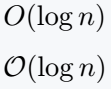In most cases, you will notice the use of O symbols instead ofin various books or documents.

 Symbol Big O notation Type asymptotic Package mismath, physics Commands O(arg), \mathcal{O}(arg) Example O(log n) → O(log n)
\documentclass{article}
\begin{document}
$O(n^2)$
$O(n^c)$
$O(g(x))$
$O(\max(g_1,g_2))$
\end{document}

Output :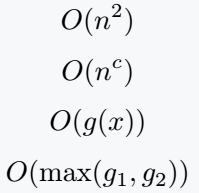You have always noticed that backslash is used before the command name. But, in this case, there is no need.

\mathcal{o} will return big o. However, the top-left side of the capital o will remain open in terms of shape.

\documentclass{article}
\begin{document}
$\mathcal{O}(\log n)$
$\mathcal{O}(\log n^c)$
$\mathcal{O}(n\log n)$
$\mathcal{O}(n!)$
\end{document}

Output :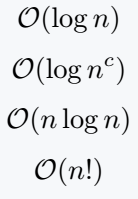Above two methods do not require any package. However, below we will learn two more ways to represent this symbol with the help of mismath and physics package.

## \bigo and \big0 commands in mismath package

Use of mismath package is unknown to many. But, even then, \bigo and \bigO commands can denote this asymptotic notation.

\documentclass{article}
\usepackage{mismath}
\begin{document}
$\bigo(m^n)$
$\bigo(1)$
$\bigO(n^3)$
$\bigO(2^n)$
\end{document}

Output :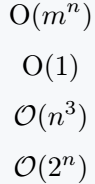Little o is used as an asymptotic notation like big o. For example

\documentclass{article}
\usepackage{mismath}
\begin{document}
$f(n)=\lito(g(n))$
$f\in \lito(g)$
$\lito \le f(n) <c*g(n)$
\end{document}

Output :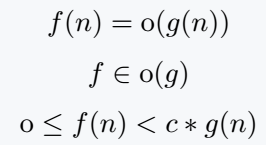## Physics package for \order{arg} command

The advantage of \order command is that the size of symbol will increase and decrease according to the size of the argument.

\documentclass{article}
\usepackage{physics}
\begin{document}
$\order{<n}$
$\order{c^n}$
$\order{n\log n}$
$\order{\order{h(n)}}$
$\order{\frac{n}{k}}$
\end{document}

Output :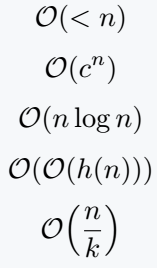If you want to manually increase the size of the parenthesis, you can use the \big on the right side of the \order command.

\documentclass{article}
\usepackage{physics}
\begin{document}
$\order\big{\log_b n}$
$\order\big{\log_c n}$
$\order\big{f(x)}$
$\order\Big{\frac{n}{q}} , \order\bigg{\frac{n_i}{q_k}} , \order\Bigg{\frac{n_{ij}}{q_{kj}}}$
\end{document}

Output :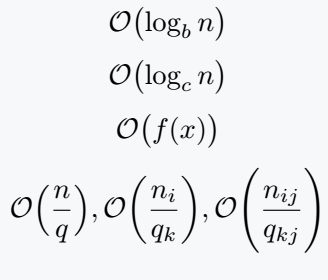One thing to keep in mind is that the size of the symbol is fixed by using the * sign with each command in the physics package.

\documentclass{article}
\usepackage{physics}
\begin{document}
$\order*{n^{\frac{5}{2}}}$
$\order*{\frac{n}{k}}$
$\order*{f(x)}$
\end{document}

Output :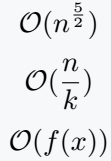#### Md Jidan Mondal

LaTeX expert with over 10 years of experience in document preparation and typesetting. Specializes in creating professional documents, reports, and presentations using LaTeX.